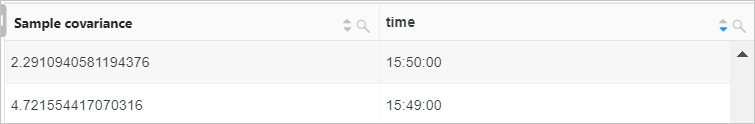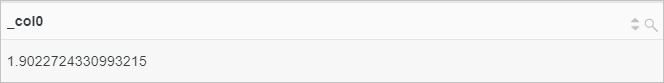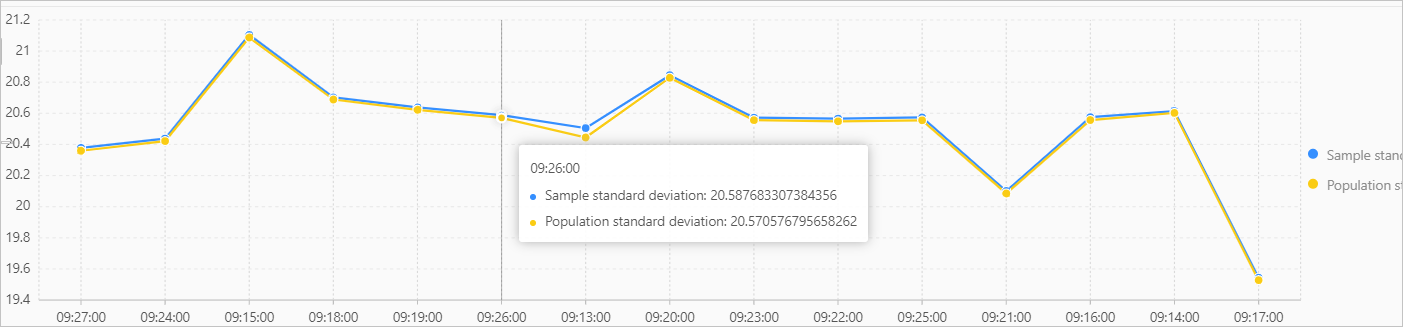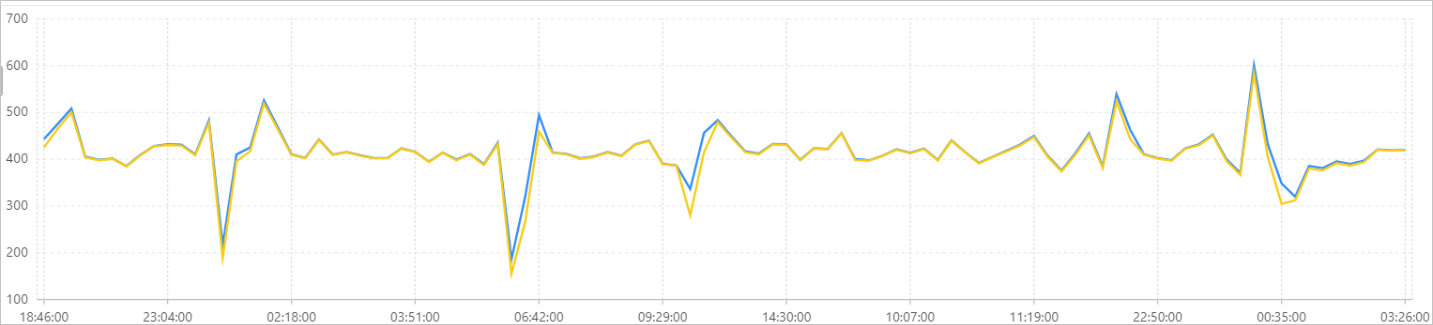This topic describes the syntax of mathematical statistics functions. This topic also provides examples on how to use the functions.

Function Syntax Description
corr function corr(x,y) Returns the coefficient of correlation between x and y. The return value is in the range of [0,1].
covar_pop function covar_pop(x,y) Returns the population covariance of x and y.
covar_samp function covar_samp(x,y) Returns the sample covariance of x and y.
regr_intercept function regr_intercept(y,x) Returns the y-intercept of the line for the linear equation that is determined by the (x,y) pair.
regr_slope function regr_slope(y,x) Returns the slope of the line for the linear equation that is determined by the (x,y) pair.
stddev function stddev(x) Returns the sample standard deviation of x. This function is equivalent to the stddev_samp function.
stddev_samp function stddev_samp(x) Returns the sample standard deviation of x.
stddev_pop function stddev_pop(x) Returns the population standard deviation of x.
variance function variance(x) Returns the sample variance of x. This function is equivalent to the var_samp function.
var_samp function var_samp(x) Returns the sample variance of x.
var_pop function var_pop(x) Returns the population variance of x.

## corr function

The corr function returns the coefficient of correlation between x and y. A larger return value indicates a higher correlation.

### Syntax

``corr(x,y)``

### Parameters

Parameter Description
x The value of this parameter is of the double type.
y The value of this parameter is of the double type.

### Return value type

The double type. The return value is in the range of [0,1].

### Examples

Calculate the coefficient of correlation between the values of the request_length and request_time fields.

• Query statement
``* | SELECT corr(request_length,request_time)``
• Query and analysis results## covar_pop function

The covar_pop function returns the population covariance of x and y.

### Syntax

``covar_pop(x,y)``

### Parameters

Parameter Description
x The value of this parameter is of the double type.
y The value of this parameter is of the double type.

The double type.

### Examples

Calculate the population covariance of pretax profits and pretax turnovers in each minute.

• Query statement
``````*|
SELECT
covar_pop(PretaxGrossAmount, PretaxAmount) AS "Population covariance",
time_series(__time__, '1m', '%H:%i:%s', '0') AS time
GROUP BY
time``````
• Query and analysis results## covar_samp function

The covar_samp function returns the sample covariance of x and y.

### Syntax

``covar_samp(x,y)``

### Parameters

Parameter Description
x The value of this parameter is of the double type.
y The value of this parameter is of the double type.

The double type.

### Examples

Calculate the sample covariance of pretax profits and pretax turnovers in each minute.

• Query statement
``````*|
SELECT
covar_samp(PretaxGrossAmount, PretaxAmount) AS "Sample covariance",
time_series(__time__, '1m', '%H:%i:%s', '0') AS time
GROUP BY
time``````
• Query and analysis results## regr_intercept function

The regr_intercept function returns the y-intercept of the line for the linear equation that is determined by the (x,y) pair. x is the dependent value. y is the independent value.

### Syntax

``regr_intercept(y,x)``

### Parameters

Parameter Description
y The value of this parameter is of the double type.
x The value of this parameter is of the double type.

The double type.

### Examples

Calculate the y-intercept of the line for the linear equation that is determined by the values of the request_time and request_length fields.

• Query statement
``* | SELECT regr_intercept(request_length,request_time)``
• Query and analysis results## regr_slope function

The regr_slope function returns the slope of the line for the linear equation that is determined by the (x,y) pair. x is the dependent value. y is the independent value.

### Syntax

``regr_slope(y,x)``

### Parameters

Parameter Description
y The value of this parameter is of the double type.
x The value of this parameter is of the double type.

The double type.

### Examples

Calculate the slope of the line for the linear equation that is determined by the values of the request_time and request_length fields.

• Query statement
``* | SELECT regr_slope(request_length,request_time)``
• Query and analysis results## stddev function

The stddev function returns the sample standard deviation of x. This function is equivalent to the stddev_samp function.

### Syntax

``stddev(x)``

### Parameters

Parameter Description
x The value of this parameter is of the double or bigint type.

The double type.

### Examples

Calculate the sample standard deviation and population standard deviation of pretax incomes and display the calculated values in a line chart.

• Query statement
``````* |
SELECT
stddev(PretaxGrossAmount) as "Sample standard deviation",
stddev_pop(PretaxGrossAmount) as "Population standard deviation",
time_series(__time__, '1m', '%H:%i:%s', '0') AS time
GROUP BY
time``````
• Query and analysis results## stddev_samp function

The stddev_samp function returns the sample standard deviation of x.

### Syntax

``stddev_samp(x)``

### Parameters

Parameter Description
x The value of this parameter is of the double or bigint type.

The double type.

### Examples

Calculate the sample standard deviation and population standard deviation of pretax incomes and display the calculated values in a line chart.

• Query statement
``````* |
SELECT
stddev_samp(PretaxGrossAmount) as "Sample standard deviation",
stddev_pop(PretaxGrossAmount) as "Population standard deviation",
time_series(__time__, '1m', '%H:%i:%s', '0') AS time
GROUP BY
time``````
• Query and analysis results## stddev_pop function

The stddev_pop function returns the population standard deviation of x.

### Syntax

``stddev_pop(x)``

### Parameters

Parameter Description
x The value of this parameter is of the double or bigint type.

The double type.

### Examples

Calculate the sample standard deviation and population standard deviation of pretax incomes and display the calculated values in a line chart.

• Query statement
``````* |
SELECT
stddev(PretaxGrossAmount) as "Sample standard deviation",
stddev_pop(PretaxGrossAmount) as "Population standard deviation",
time_series(__time__, '1m', '%H:%i:%s', '0') AS time
GROUP BY
time``````
• Query and analysis results## variance function

The variance function returns the sample variance of x. This function is equivalent to the var_samp function.

### Syntax

``variance(x)``

### Parameters

Parameter Description
x The value of this parameter is of the double or bigint type.

The double type.

### Examples

Calculate the sample variance and population variance of pretax incomes and display the calculated values in a line chart.

• Query statement
``````* |
SELECT
variance(PretaxGrossAmount) as "Sample variance",
var_pop(PretaxGrossAmount) as "Population variance",
time_series(__time__, '1m', '%H:%i:%s', '0') as time
GROUP BY
time``````
• Query and analysis results## var_samp function

The var_samp function returns the sample variance of x.

### Syntax

``var_samp(x)``

### Parameters

Parameter Description
x The value of this parameter is of the double or bigint type.

The double type.

### Examples

Calculate the sample variance and population variance of pretax incomes and display the calculated values in a line chart.

• Query statement
``````* |
SELECT
var_samp(PretaxGrossAmount) as "Sample variance",
var_pop(PretaxGrossAmount) as "Population variance",
time_series(__time__, '1m', '%H:%i:%s', '0') as time
GROUP BY
time``````
• Query and analysis results## var_pop function

The var_pop function returns the population variance of x.

### Syntax

``var_pop(x)``

### Parameters

Parameter Description
x The value of this parameter is of the double or bigint type.

The double type.

### Examples

Calculate the sample variance and population variance of pretax incomes and display the calculated values in a line chart.

• Query statement
``````* |
SELECT
variance(PretaxGrossAmount) as "Sample variance",
var_pop(PretaxGrossAmount) as "Population variance",
time_series(__time__, '1m', '%H:%i:%s', '0') as time
GROUP BY
time``````
• Query and analysis results